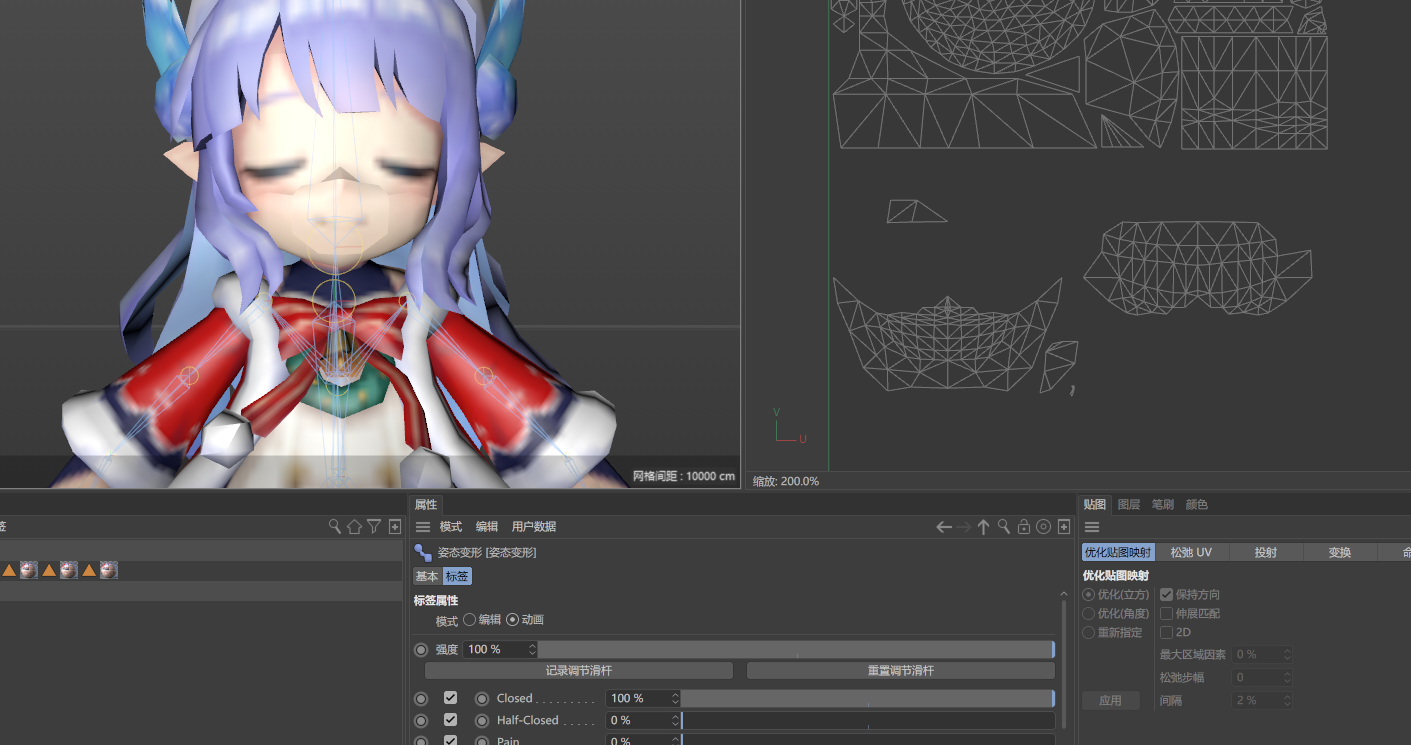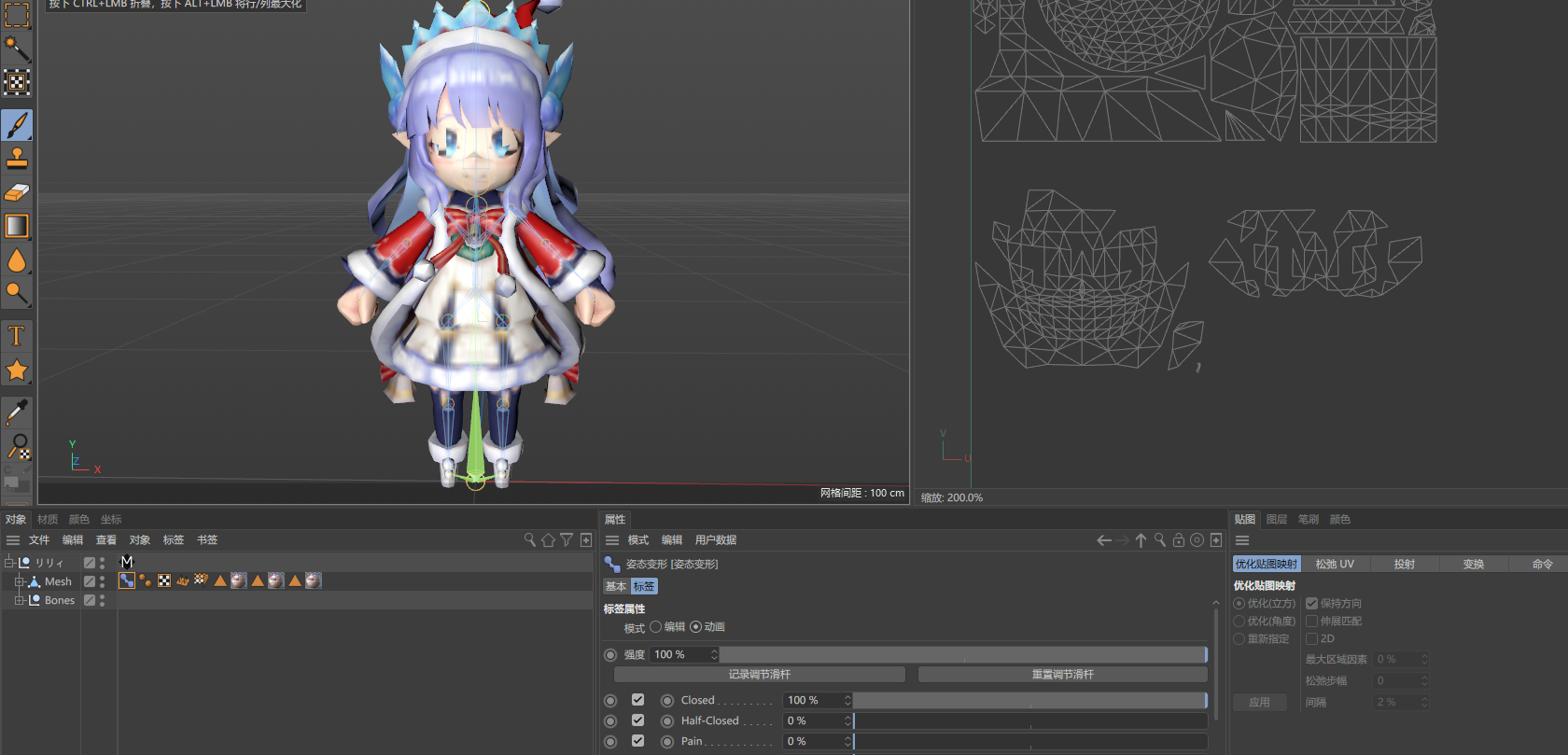# SOLVEDHow to use UV morph node

Hi,
I want to use the morph node to set some UV morphs. I didn't find the setting for setting UV morph information on the C++ SDK Documentation, only how to read it.
The UV morph information I have is for each vertex, and I noticed that the UV morph node can only set a UVWStruct for each polygon. Is there any way to set it for each vertex?

Thanks,
AiMiDi

Hi,
Thank you so much for your suggestion. I changed my code to iterate each surface. It works very well. Thank you for your help, which solved my problem.Thanks,
Manuel

Hi,

You are talking about the Morph tag righ?
The only way is to use the UVWStruct because each vertex can have as many UVs coordinates as the vertex have polygon neighbor.
If the vertex is used by 4 polygons, you can have 4 uv coordinates for that vertex.

Cheers,
Manuel

I tried to set the UV morph through each surface, but it did not work according to my assumptions. Here I want to move the entire uv, but only part of the uv is moved. What is wrong.

``````    Int32 offset_count;//The UV morph vertex count.
maxon::HashMap<Int32, Vector> vertex_floats_map; //This map stores the UV transformation of each vertex.

//Create the basic morph
morph_tag->ExitEdit(doc, true);
if (morph == nullptr)
morph->SetName(morph_data->morph_name_local);
morph->Store(doc, morph_tag, CAMORPH_DATA_FLAGS::UV);

CAMorphNode *morph_node = morph->GetFirst();
morph->SetMode(doc, morph_tag, CAMORPH_MODE_FLAGS::ALL | CAMORPH_MODE_FLAGS::EXPAND, CAMORPH_MODE::REL);
maxon::ParallelFor::Dynamic(0, offset_count, [&morph_node, &vertex_surface_map, &vertex_floats_map](const Int32 pointIndex)
{
Int surface_index; //The surface index corresponding to the vertex, the acquisition process is omitted.
CPolygon *surface = surface_data.Read()->operator[](surface_index); //Get the corresponding surface.
UVWStruct uvw;
morph_node->GetUV(0, surface_index, uvw);
auto vertex_a_ptr = vertex_floats_map.Find(surface->a);
if (vertex_a_ptr != nullptr)
{
uvw.c = vertex_a_ptr->GetValue();
}
auto vertex_b_ptr = vertex_floats_map.Find(surface->b);
if (vertex_b_ptr != nullptr)
{
uvw.b = vertex_b_ptr->GetValue();
}
auto vertex_c_ptr = vertex_floats_map.Find(surface->c);
if (vertex_c_ptr != nullptr)
{
uvw.a = vertex_c_ptr->GetValue();
}
morph_node->SetUV(0, surface_index, uvw);
});
morph->SetMode(doc, morph_tag, CAMORPH_MODE_FLAGS::ALL | CAMORPH_MODE_FLAGS::COLLAPSE, CAMORPH_MODE::AUTO);
morph_tag->UpdateMorphs();
morph_tag->Message(MSG_UPDATE);
morph->SetStrength(0);
morph_tag->SetParameter(DescID(ID_CA_POSE_MODE), ID_CA_POSE_MODE_ANIMATE, DESCFLAGS_SET::NONE);
``````

Please see here for the complete.Thanks,
AiMiDi

Hi,

It's a bit complexe to understand what's going wrong here, specially without having an example to reproduce. You say that some parts are moving but not everything. That mean you got the logic right but not the code. i don't think providing an example will help you too much.

My suggestion is to simplify your code as much as possible or work on a simplest example.
I would also iterate over all polygon and not over all vertices. (but if you are mor confortable with vertices, keep it).

Cheers,
Manuel

Hi,
Thank you so much for your suggestion. I changed my code to iterate each surface. It works very well. Thank you for your help, which solved my problem.Thanks,
Manuel